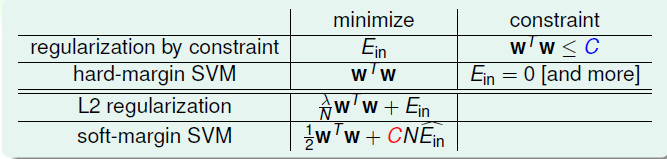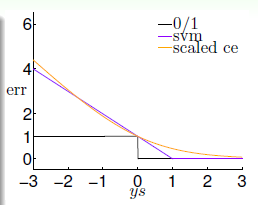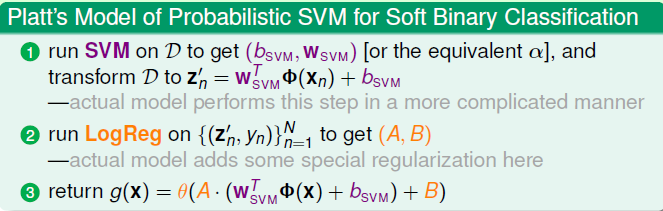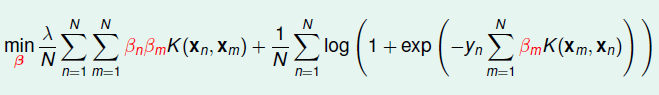# Kernel Logistic Regression

## SVM 与 正则化

\begin{align}\min\limits_{b,\mathbf{w}, \xi} \quad &\frac{1}{2} \mathbf{w}^T\mathbf{w} + C \cdot \sum\limits_{n=1}^{N}\xi_n \\ s.t. \quad & y_n(\mathbf{w}^T\mathbf{z}^n+b) \geq 1-\xi_n, for \ all\ n \end{align}

\begin{align} & \min\limits_{b,\mathbf w}\left({1\over 2}\mathbf w^T\mathbf w + C\sum_{n=1}^N\max\left(1-y_n\left(\mathbf w^T\mathbf z_n + b\right), 0\right)\right) \\ \Leftrightarrow &\min\limits_{b,w}\left({1\over 2}\mathbf w^T\mathbf w + C \cdot \sum \widehat{err} \right) \end{align}

$\min\limits_{b,\mathbf w}\left({\lambda\over N}\mathbf w^T\mathbf w + {1\over N}\sum\mbox{err}\right)$

$L_2$中，通过最小化$E_{in}$的同时控制$\mathbf{w}$的大小，防止模型过度复杂。从正则化的角度来看的话，Soft-Margin 的SVM其实就属于一种正则化模型，如下的对比:## SVM With Soft Binary Classification

\begin{align} PLA: \quad & err_{0/1}(s, y) = [[ys \leq 0]] \\ LR: \quad & err_{SCE}(s,y) = \log_{2}(1+\exp(-ys)) \\ SVM: \quad & \widehat{err}_{SVM}(y,s) = \max(1-ys,0)\end{align}• 第一种: 首先使用SVM得到$(b_{SVM}, \mathbf w_{SVM})$，然后直接使用sigmod函数计算概率，得到模型: $g(x) = (W^T_{SVM} x + b_{SVM})$
• 第二种: 使用SVM得到$(b_{SVM}, \mathbf w_{SVM})$，然后作为LR的初始$\mathbf w_0$,再运行LR得到最终的模型

$g(\mathbf x) = \theta(A \cdot (\mathbf w^T_{SVM}\phi(\mathbf x)+b_{SVM}) + B)$$\theta\left( \sum\limits_{SV}A \alpha_ny_nK(\mathbf x_n, \mathbf x) + Ab_{SVM} +B\right)$

## Kernel Logistic Regression(核逻辑回归)

$\mathbf w_{*}^{T} \mathbf z = \sum\beta_n\mathbf z_n \mathbf z = \sum \beta_n K(\mathbf x_n, \mathbf x)$

• SVM: $\mathbf w_{svm} = \sum (\alpha_ny_n)\mathbf z_n$，其中$\alpha$是对偶问题中的拉格朗日乘子
• PLA: $\mathbf w_{PLA} = \sum (\alpha_ny_n)\mathbf z_n$，这里的$\alpha$则是在修正$\mathbf w$的过程中，$\mathbf z_n$这个点被分错误的次数
• LR: $\mathbf w_{LR} = \sum (\alpha_ny_n)\mathbf z_n$，这里的$\alpha_n$可以理解为在用梯度下降法更新$\mathbf w$的步长
• ...

$\min\limits_{\mathbf w} \quad {\lambda \over N}\mathbf w^T \mathbf w + {1 \over N}\sum\limits_{n=1}^{N}err(y_n, \mathbf w^T \mathbf z_n)$

$\min_{\mathbf w} \quad {\lambda\over N}\mathbf w^T\mathbf w+{1\over N}\sum_{n=1}^N\log\left(1+\exp\left(-y_n\mathbf w^T\mathbf z_n\right)\right)$